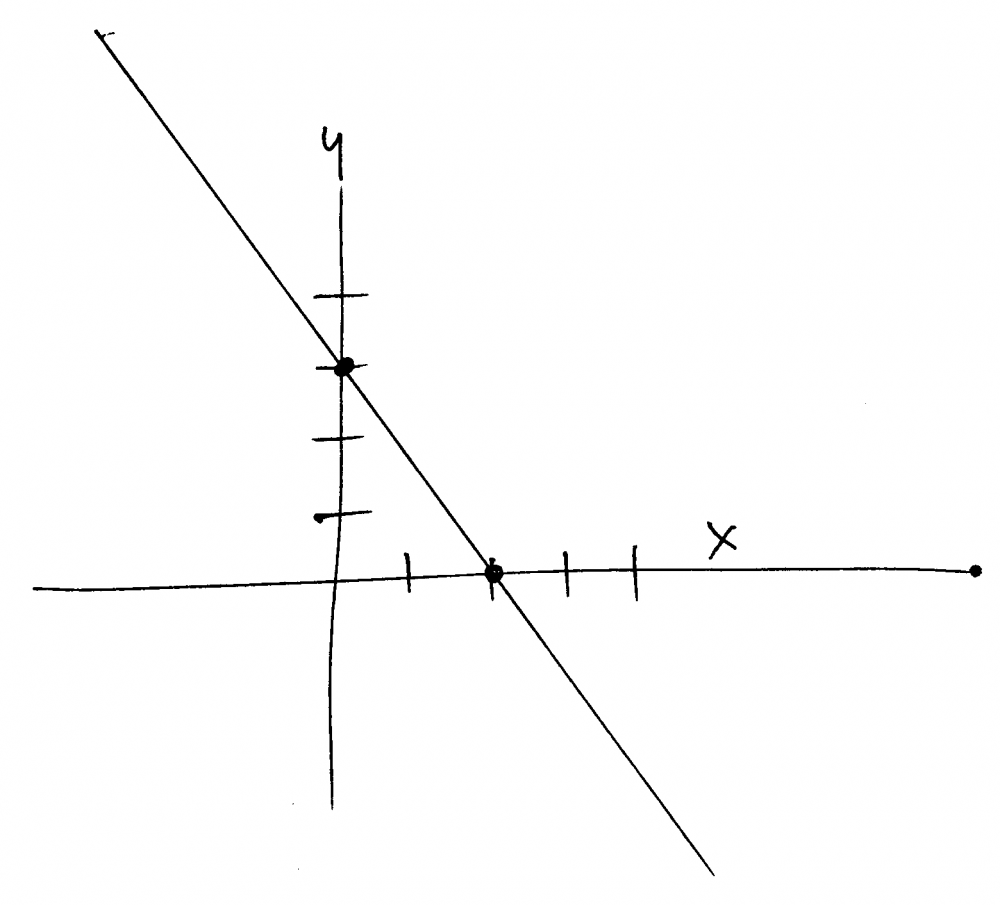2y=3x=6 Graph Equation, Can You Answer It?

You could do this a couple different ways.

1:  put any number into y, and solve for x.  plot that point on a graph.  do the same for a different value of y, and solve for x.  plot it.  do a couple more just to make sure they're all on the same line.  If one isn't, that's probably a math error.

2:  convert the equation to y=mx+b form.  in that case, the y intercept is found by setting x = 0.  Similarly, you could find another point, and plot it with a different value of y, but since you already have the y intercept you can make a line using the slope (if that's easier).  once you put it into y= mx+b form, use m as your slope.  but check a new point from your equation against the graph to verify you got the slope right.

With linear equations, two points determine a line, but using more points will help you verify they're correct.

thanked the writer.
Anonymous commented
very detail....sometimes showing step by step will help too.
Anonymous commented
I'm thinking there's an error and the equation is 2y-3y=6 or something like that. It's not likely to have two equalities, but if it does there's only one solution, a single point.
As Nic7320 says, two points determine a line. For an equation such as this, two points are fairly easy to determine.

X-intercept
Choose y=0
2(0)+3x=6
3x=6
x=6/3=2   one (x, y) point is (2, 0)

Y-intercept
Choose x=0
2y+3(0)=6
2y=6
y=6/2=3   another (x, y) point is (0, 3)

Locate the first point on the x-axis and the second point on the y-axis. Draw a line through them and beyond on either side and you have graphed the equation.thanked the writer.
2y=3x
thanked the writer.
Y=2/3x+6
thanked the writer.
2y=3x=6
thanked the writer.